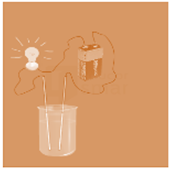# Acid, Base And Salt

15 Questions | Total Attempts: 7874SettingsTime: 30 Minute

• 1.
In which one of the following solutions the bulb will not glow when electric current passed through it?
• A.

Tap water

• B.

Hydrochloric acid

• C.

Glucose solution

• D.

Dilute sulphuric acid

• 2.
Guard tube containing anhydrous calcium chloride is used to
• A.

Absorb water from air

• B.

Absorb colored component

• C.

Absorb N2 from air

• D.

Absorb O2 from air

• 3.
Which of the following statements is correct about an aqueous solution of an acid and of a base? (i) Higher the pH, stronger the acid (ii) Higher the pH, weaker the acid (iii) Lower the pH, stronger the base (iv) Lower the pH, weaker the base
• A.

(i) and (iii)

• B.

(ii) and (iii)

• C.

(i) and (iv)

• D.

(ii) and (iv)

• 4.
Which of the following phenomena occur, when a small amount of acid is added to water? i. Ionisation ii. Neutralisation iii. Dilution iv. Salt formation
• A.

(i) and (ii)

• B.

(i) and (iii)

• C.

(ii) and (iii)

• D.

(ii) and (iv)

• 5.
In an attempt to demonstrate electrical conductivity through an electrolyte, the following apparatus (Given Figure) was set up. Which among the following statement(s) is(are) correct? i. Bulb will not glow because electrolyte is not acidic ii. Bulb will glow because NaOH is a strong base and furnishes ions for conduction. iii. Bulb will not glow because it depends upon the type of electrolytic solution
• A.

(i) and (iii)

• B.

(ii) and (i)

• C.

(ii) only

• D.

(iii) and (ii)

• 6.
Identify the correct representation of reaction occurring during chloralkali process
• A.

2NaCl(l) + 2H2O(l) → 2NaOH(l) + Cl2(g) + H2(g

• B.

2NaCl(aq) + 2H2O(aq) → 2NaOH(aq) + Cl2(g) + H2(g)

• C.

2NaCl(aq) + 2H2O(l) → 2NaOH(aq) + Cl2(aq) + H2(aq)

• D.

2NaCl (aq) + 2H2O (l) → 2NaOH (aq) + Cl2(g) + H2(g)

• 7.
Which of the following is(are) true when HCl (g) is passed through water? i. It does not ionise in the solution as it is a covalent compound. ii. It ionises in the solution iii. It gives both hydrogen and hydroxyl ion in the solution iv.  It forms hydronium ion in the solution due to the combination of hydrogen ion with water molecule
• A.

(i) only

• B.

(iii) only

• C.

(ii) and (iv)

• D.

(iii) and (iv)

• 8.
One of the following does not inject and acidic liquid into the skin through its sting. This us :
• A.

Honey bee

• B.

Ant

• C.

Wasp

• D.

Nettle leaf hair

• 9.
10 ml of a solution of NaOH is found to be completely neutralized by 8 ml of a given solution of HCl. If we take 20 ml of the same solution of NaOH, the amount of HCL (the same solution as before) required to neutralize it will be –
• A.

4 ml

• B.

8 ml

• C.

12 ml

• D.

16 ml

• 10.
In the following reaction, identify the salt formed NH4OH(aq)+H2SO4(aqà----+2H2O(I)
• A.

NH4NO3

• B.

(NH4)2SO4

• C.

(NH4)3PO4

• D.

(NH4)2S

• 11.
Which of the following statements is not correct?
• A.

All metal carbonates react with acid to give a salt, water and carbon dioxide

• B.

All metal oxides react with water to give salt and acid

• C.

Some metals react with acids to give salt and hydrogen

• D.

Some non metal oxides react with water to form an acid

• 12.
The following data were collected at the endpoint of a titration performed to find the molarity of an HCl solution. Volume of acid (HCl) used = 14.4 mL Volume of base (NaOH) used = 22.4 mL Molarity of standard base (NaOH) = 0.20 M What is the molarity of the acid solution?
• A.

1.6 M

• B.

0.64 M

• C.

0.31M

• D.

0.13M

• 13.
When HCl(aq) is exactly neutralized by NaOH(aq), the hydrogen ion concentration in the resulting mixture is
• A.

Always less than the concentration of the hydroxide ions

• B.

Always greater than the concentration of the hydroxide ions

• C.

Always equal than the concentration of the hydroxide ions

• D.

Sometimes greater and sometimes less than the concentration of the hydroxide ionsz

• 14.
A student wishes to prepare approximately 100 milliliters of an aqueous solution of 6M HCl using 12 M HCl. Which procedure is correct?
• A.

Adding 50 mL of 12 M HCl to 50 mL of water while stirring the mixture steadily

• B.

Adding 50 mL of 12 M HCl to 50 mL of water and then stirring the mixture steadily

• C.

Adding 50 mL of water to 50 mL of 12 M HCl while stirring the mixture steadily

• D.

Adding 50 mL of water to 50 mL of 12 M HCl and then stirring the mixture steadily

• 15.
Given the equation: H+ + OH- <-> H2O which type of reaction does the equation represent?
• A.

Esterification

• B.

Decomposition

• C.

Hydrolysis

• D.

Neutralization

Related TopicsBack to top